#### You may also like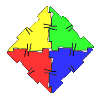### Polydron

This activity investigates how you might make squares and pentominoes from Polydron.### Eight Dominoes

Using the 8 dominoes make a square where each of the columns and rows adds up to 8### Prime Magic

Place the numbers 1, 2, 3,..., 9 one on each square of a 3 by 3 grid so that all the rows and columns add up to a prime number. How many different solutions can you find?

# Symmetry Challenge

##### Age 7 to 11 Challenge Level:

Christina from Marborough Primary, London has given this one some thought and made the sensible suggestion of trying to find all the symmetrical patterns with one coloured square, then with two squares, then three, then four. That's just what Tom has done below.

Tom was very careful to make sure he found them all. First, he looked for patterns with no coloured squares. Of course, there's only one of those: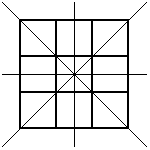Then he looked for patterns with one coloured square. He decided to count two patterns as the same if they were just rotations of each other, as otherwise there would be too many. Here are the patterns he found: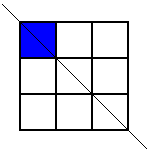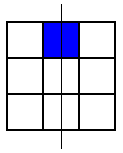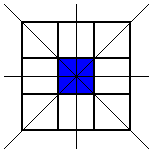Next he looked for patterns with two coloured squares. He had to be a bit more careful here, to make sure that he didn't miss any. First of all, he coloured in the top left square. Then he wondered whether he could find any patterns with this square coloured where the line of symmetry was vertical. He found this one: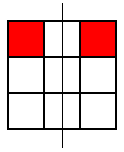but that was the only one. Then he looked for patterns with this square coloured where the line of symmetry was horizontal, but he came up with the same one again (rotated, of course). Then he looked for ones where the line of symmetry was diagonal. Here are the two patterns he found: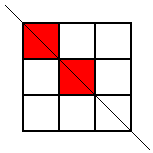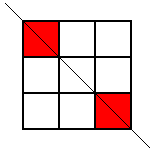These were the only symmetrical patterns with a corner square shaded. Next he shaded the top centre square and looked for patterns with a vertical line of symmetry. Here's what he found: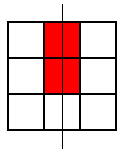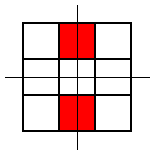He noticed that he wouldn't get any new patterns by looking for ones with horizontal lines of symmetry, so he looked for patterns with a diagonal line of symmetry. This is the only one he found: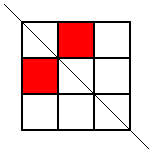He used a similar system to find all the symmetrical patterns with three or four shaded squares. Here's what he found: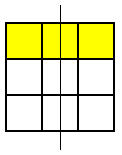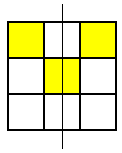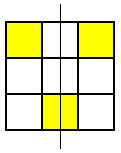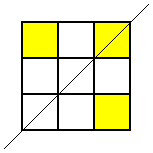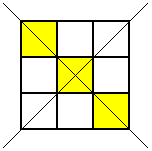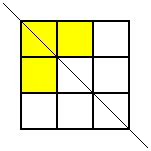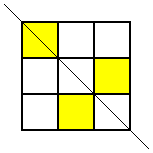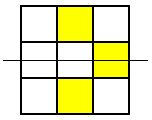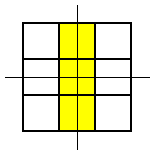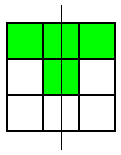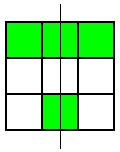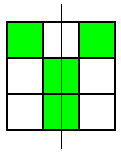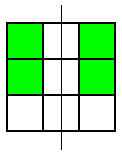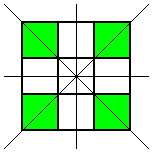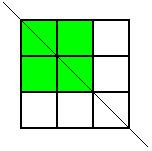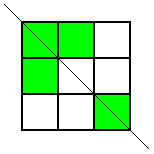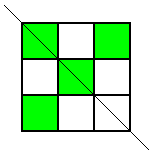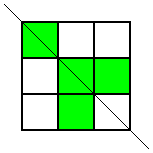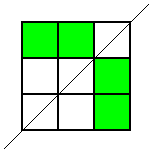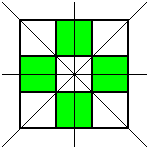Finally, Tom noticed that really these told him all of the symmetrical patterns, because he could imagine a coloured grid where the white squares were the ones that had been shaded, and these would give the patterns with five, six, seven, eight or nine shaded squares.
So Tom found 62 symmetrical patterns in all.

Children from Kellett School in Hong Kong, noticed that Tom had missed out one of the patterns.  Here is the image they sent: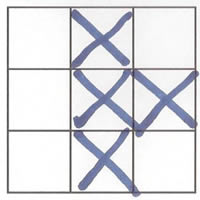So that makes a total of 64 possible solutions.

Pupils from Markham College in Peru wrote to say that they didn't think a white square with no colouring at all would count as a solution (that is the very first one that Tom includes above), so they concluded there are 63 solutions in total.